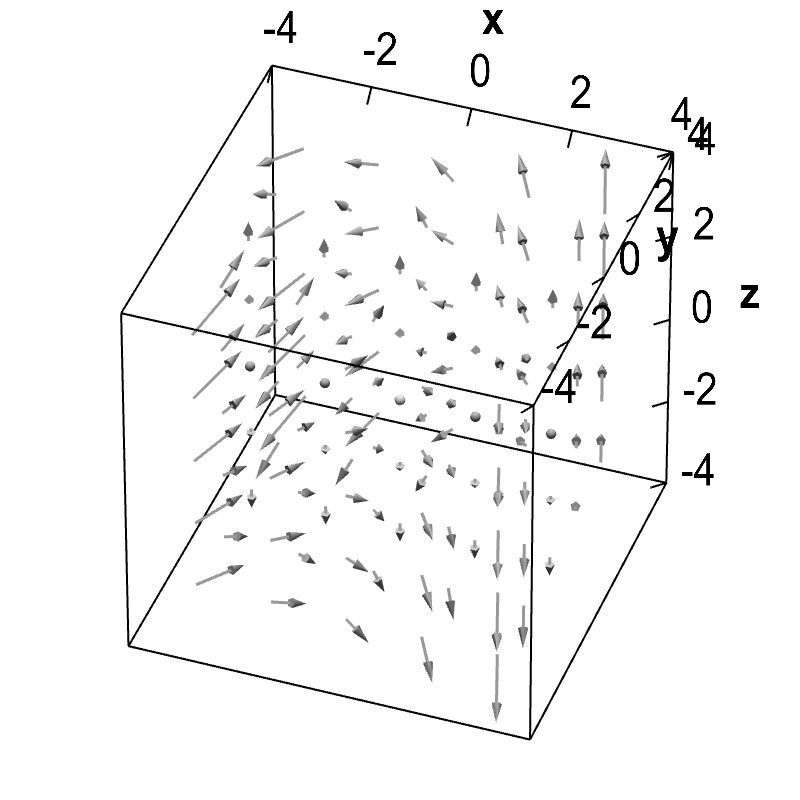# Math Insight

### Applet: Example three-dimensional vector fieldThe structure of the vector field is difficult to visualize, but rotating the graph with the mouse helps a little. This vector field has both nonzero divergence and curl.

The vector field is $\dlvf = (-y, xy,z)$. Its divergence is $\div \dlvf(x,y,z) = x+1$, and its curl is $\curl \dlvf = (0,0,y+1)$